Courses

Test: Network Theory

25 Questions MCQ Test GATE ECE (Electronics) 2022 Mock Test Series | Test: Network Theory

Description
Attempt Test: Network Theory | 25 questions in 75 minutes | Mock test for GATE preparation | Free important questions MCQ to study GATE ECE (Electronics) 2022 Mock Test Series for GATE Exam | Download free PDF with solutions
QUESTION: 1

Unit of inductance is ________.

Solution:

The unit of inductance is Henry. Weber is the unit of magnetic flux. Tesla is the unit of flux density. Farad is the unit of capacitance.

QUESTION: 2

Which of the following is not the energy stored in a capacitor?

Solution:

Energy stored in a capacitor, E = CV2/2
Using C = Q/V
E = CV2/2 = QV/2 = Q2/2C

QUESTION: 3

Two AC sources feed a common variable resistive load as shown in figure below.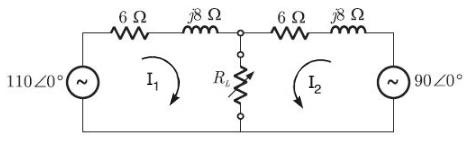The maximum power that can be transferred to a variable load RL is _______ W.

Solution: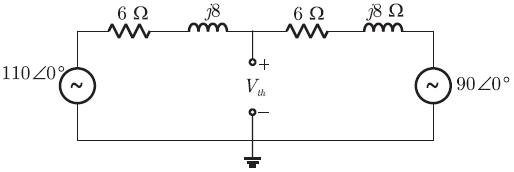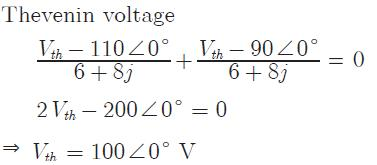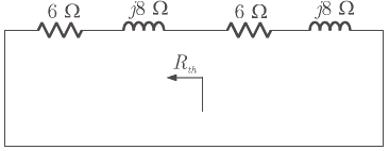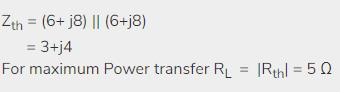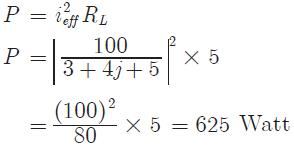QUESTION: 4

The switch of below circuit was open for long and at t = 0 it is closed. What is the final steady state voltage across the capacitor and the time constant of the circuit?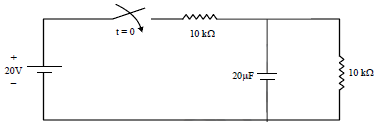Solution:

Final value = 20/2 = 10 Volt
Time constant τ= 5 × 103 × 20 × 10-6
= 0.1 sec

QUESTION: 5

The y parameter of the network given in figure is: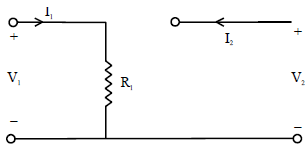Solution: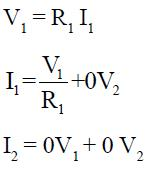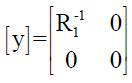QUESTION: 6

The power consumed by a coil is 300 W when connected to 30 V dc source and 108 W. When connected to a 30 V A.C. source. The reactance of the coil is:

Solution:
• In DC, inductive reactance X=0; since f=0;
• Only, Resistance exist in coil for DC,
R=(30*30/300)=3 ohm;
• In AC, Z=R+jX;
• But here in second part of question we given that real power in ac circuit is 108 watt. We can calculate current in the ac circuit:
I=√(108/3)= √36=6 amp
• This current flow through resistance of AC circuit so this is the current of AC circuit.
• So,
Z =V/I
Z=30/6=5
X=√{5^2–3^2} = 4ohm
QUESTION: 7

For a transmission line open circuit and short circuit impedances are 20 Ω and 5 Ω. Then characteristic impedance is:

Solution:
• The characteristic impedance is the geometric mean of the short and open circuit impedance.
• It is given by Zo2 = Zsc Zoc. On substituting Zoc = 20 and Zsc = 5, we get Zo2 = 20 X 5 = 100.
• Thus Zo = 10 ohm.
QUESTION: 8

A human nerve cell has an open circuit voltage of 80 mV and it can deliver a current of 5 nA through a 6 MΩ load. What is the maximum power available from the cell?

Solution: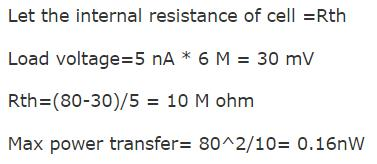QUESTION: 9

The Thevenin impedance across the terminal AB of the given network is: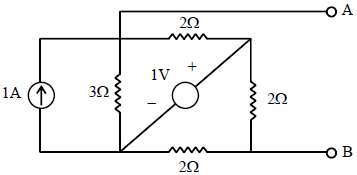Solution: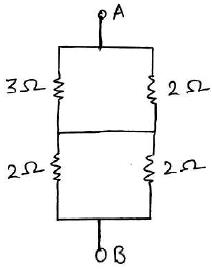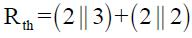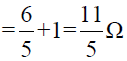QUESTION: 10

The circuit shown in the figure Ris variable between 3 and 30 ohms RL being fixed.
Assertion (A): For Maximum power transfer across the terminals AB, Rg = 3?
Reason (R): When Maximum power transfer occurs RL = |Zg|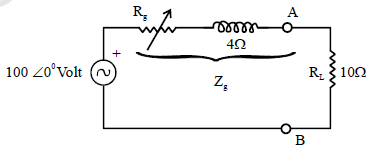Solution: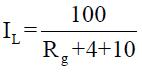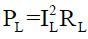• PL is Maximum when IL is Maximum
• IL is Maximum when Rg in Minimum = 3Ω
• Thus A is True
• For Maximum power Transfer KL= |Zs|
• But here Rg is variable, so it should be low so R is True but not the correct explanation of A.
QUESTION: 11

A line to neutral voltage is 10∠150V for a balanced three phase star connected load with phase sequence ABC. The voltage of line B with respect to line C is given by:

Solution: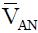= 10 ∠150 Volt
Phase sequence is ABC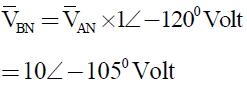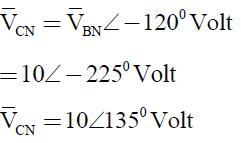Line Voltage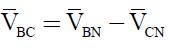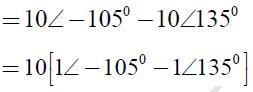= 10[1∠ - 1200 x 1∠15 - 1∠1200 x 1∠15]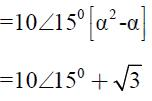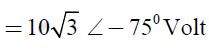*Answer can only contain numeric values
QUESTION: 12

Determine Maximum power consumed in the load resistance R_____ (in watts)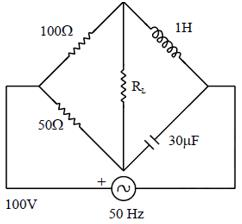(Important - Enter only the numerical value in the answer)

Solution:

ω = 2πf = 2π x 50 = 100π
XL = ωL , Xc = 1/ωc
Thevenin equivalent across RL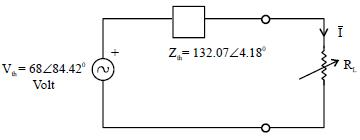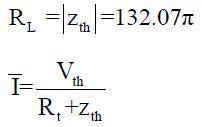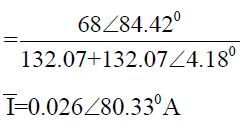PMax = ± 2RL = (0.026)2 x 132.07
PMax = 8.92 Watt

QUESTION: 13

A series R-L-C circuit resonates at 4Hz it provides 0.707 lag P.F. at 8 Hz. The frequency at which it gives 0.707 lead P.F is?

Solution:

f0 = 4 Hz,
Upper cut off frequency
f2 = 8Hz
lower cutoff f1 = ?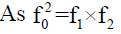(4)2 = f1 x 8
16 = f1 x 8
f1 = 2Hz

QUESTION: 14

In series RLC circuit, the voltage across capacitor and inductor are ______ with each other.

Solution:

In series RLC circuit, the voltage across capacitor and inductor are 180⁰ out of phase with each other. The frequency at which the resonance occurs is called resonant frequency.

QUESTION: 15

A series R-L-C circuit has a resonance frequency of 1kHz and a quality factor Q = 100. If each of R, L and C is doubled from its original value then new Q of the circuit is:

Solution: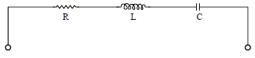Quality factor: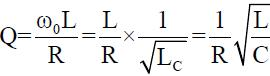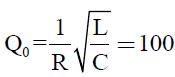if R, L, C double:
R' = 2R, L' = 2L, C' = 2C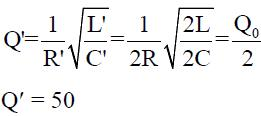QUESTION: 16

A 25 Ω resistor has a voltage of 150 sin377 t. Find the corresponding power.

Solution: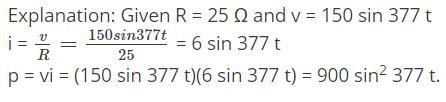QUESTION: 17

Consider the following function:

1.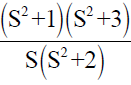2.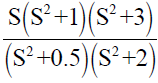3.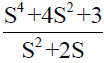4.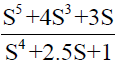Which of the above function are LC driving point impedances?

Solution:

Only (1) and (2) are L-C driving point impedance.

QUESTION: 18

If an RC driving point impedance function Z(S) has an equal number of poles and zero’s at finite locations then:

Solution:

Let us Consider: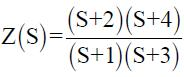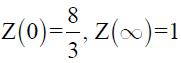QUESTION: 19

For the circuit shown below the pole’s of the driving point impedance function are at which one of the following location?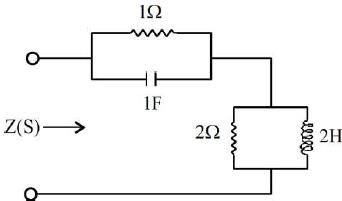Solution: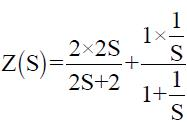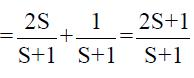A Pole at S = -1 and a zero at S = - 1/2.

QUESTION: 20

The voltage and current wave functions for an element are shown in the figures.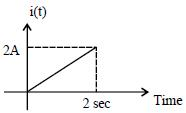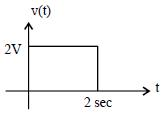The circuit element and its value are:

Solution: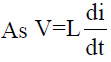For L = 2H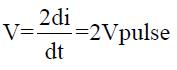So element is inductor and its value is 2H

QUESTION: 21

The steady state in the circuit shown in the given figure is reached with S open. S is closed at t = 0. The current I at t = 0+ is: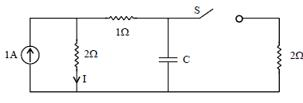Solution: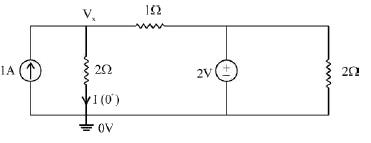KCL and node Vx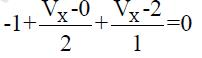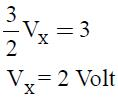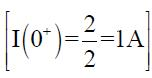QUESTION: 22

The total impedance Z(Jω) of the circuit shown below is: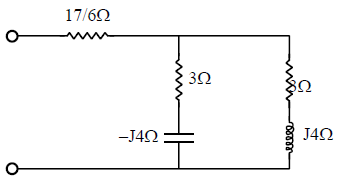Solution: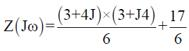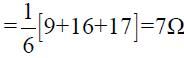QUESTION: 23

Identify which of the following is not a tree of the graph shown in figure.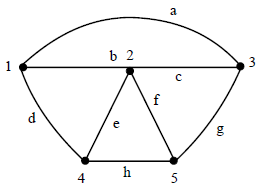Solution:

Tree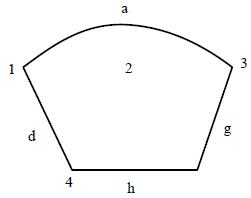QUESTION: 24

Two lamps each of 230V and 60W rating are connected in series across a single phase 230V supply. The total power consumed by the two lamps would be:

Solution: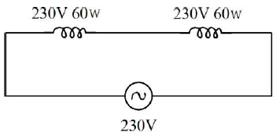Resistance of each lamp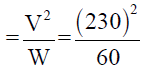Hence Total resistance offered by 2 these lamps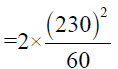So total power consumed by two lamps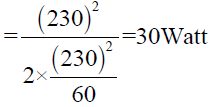QUESTION: 25

For the R-L circuit shown the current i(t) for unit step input voltage will rise to 0.63 is: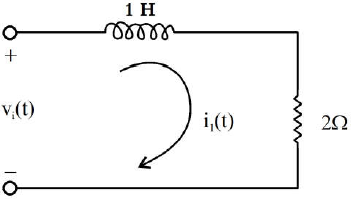Solution:

The time at which the current rise to 63% of its final value is Time constant,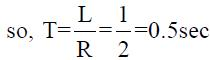Use Code STAYHOME200 and get INR 200 additional OFF Use Coupon Code

Track your progress, build streaks, highlight & save important lessons and more!

Similar ContentRelated tests